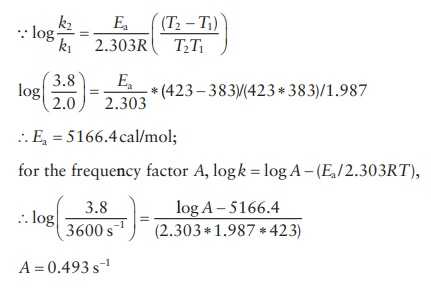| Home | | Pharmaceutical Drugs and Dosage | | Pharmaceutical Industrial Management |

## Chapter: Pharmaceutical Drugs and Dosage: Chemical kinetics and stability

Pharmaceutical Drugs and Dosage: Chemical kinetics and stability - Review questions answers

Review questions

7.1 Use one or more of the choices given below for answering the follow-ing set of questions. These questions can be answered by referring to Figures 7.1 through 7.3.

i. Zero-order reaction

ii. First-order reaction

iii. Second-order reaction

A.      For which of these reaction kinetic models is the drug con-centration at any time, t, independent of the initial drug concentration?

B.      For which of these reaction kinetic models is the half-life inde-pendent of the initial drug concentration?

C.      For which of these reaction kinetic models is the rate of drug degradation independent of the initial drug concentration?

D.      Which of these reaction kinetic models would show an expo-nential decline in drug concentration over a period of time?

E.       Which of these reaction kinetic models would give a straight line when concentration is plotted against time?

F.       Which of these reaction kinetic models would give a straight line when the inverse of concentration is plotted against time?

G.      Which of these reaction kinetic models would give a straight line when logarithm of concentration is plotted against time?

H.      For which of these reaction kinetic models is the drug con-centration at any time t (and half-life) would be greater when higher initial concentration is used (i.e., directly proportional to the initial drug concentration)?

I.          For which of these reaction kinetic models is the drug con-centration at any time t (and half-life) would be lower when higher initial concentration is used (i.e., inversely proportional to the initial drug concentration)?7.2 Which equation is used to predict the stability of a drug at room tem-perature from experiments at increased temperatures?

A.      Stokes equation

B.      Arrhenius equation

C.      Michaelis–Menten equation

D.      Henderson–Hasselbalch equation

E.       Noyes–Whitney equation

7.3 Which equation is used to predict disproportionation of a weakly acidic or a weakly basic drug as a function of pH?

A.      Stokes equation

B.      Arrhenius equation

C.      Michaelis–Menten equation

D.      Henderson–Hasselbalch equation

E.       Noyes–Whitney equation

7.4 When an acid catalyzed reaction is affected by the concentration and strength of the buffer species, it is known as:

A.      Specific-acid catalysis

B.      Specific-base catalysis

C.      General-acid catalysis

D.      General-base catalysis

7.5 In a first-order reaction involving the decomposition of hydrogen per-oxide for a period of 50 min, the concentration expressed in volume was found to be 10.6 mL from an initial concentration of 72.6 mL.

A.      Calculate k.

B.      Calculate the amount of hydrogen peroxide not decomposed after 30 min.

7.6 For a second-order reaction,

C2H5COOC2H5 + KOH C2H5COOK + C2H5OH

Diethyl acetate and potassium hydroxide were at a concentration of 0.05 M. Concentration of potassium hydroxide was observed to change by 0.0088 mol/L over a period of 35 min. Determine the rate constant, k, for the reaction and the half-life.

7.7 A formulation for an analgesic is found to degrade at 110°C (383°K), with a rate constant of k1 = 2.0 h−1 and k2 at 150°C (383°K) of 3.8 h−1. Calculate the activation energy and the frequency factor, A (R = 1.987 cal deg−1. mol−1).

7.8 The shelf life of a liquid drug is 21 days at 5°C. For approximately how long will the drug be stable at 37°C?

7.1 A. Second order

B. First order

C. Zero order

D. First order

E. Zero order

F. Second order

G. First order

H. Zero and first order

I. Second order

7.2 B. Stability at room temperature can be predicted from accelerated testing data by the Arrhenius equation: log (k2/k1) = Ea (T2T1)/ (2.303 RT2T1), where k2 and k1 are the rate constants at the abso-lute temperatures T2 and T1, respectively; R is the gas constant; and Ea is the energy of activation. Stokes’ equation is used to deter-mine the sedimentation rate of a suspension, whereas the Noyes– Whitney equation is used to determine the dissolution rate.

7.3 I.

7.4 C.

7.5 A. 2H2O2 2H2O + O2

For afirst-order reaction,

k= 2.303/t log C0/C ,

k = 2 .303/50min  *  log (72.6mL/10.6mL) = 0. 0385min1

B. C = C010 kt / 2.303 = 72. 6 10 0.0385*30/ 2.303 = 22.885mL

7.6 To calculate k, therefore diethyl acetate and potassium hydroxide have same initial concentration, x/a(ax) = kt, x = amount of reacted potassium hydroxide, a = the initial amount, k = x/(t * a * (ax)) = 0.0088 mol/L/(35 min * 0.05 mol/L * (0.05 mol/L − 0.0088 mol/L)),

k = 0. 122 L, mol1 min1

To calculate t1/2, i.e., x = 1/2a,

t = t1/ 2 = 1/2a/(ak(a. − 1/2a)) = 1/(ak) = 1/(0.05 mol/ L * 0.122 L mol−1 min−1) = 163.86 min

7.77.8 According to t 90(T2 ) = t90(T1) /Q10ΔT /10, using Q10 of 5, life at 37°C = 21days/5[(375)/10] = 0.12 day or 2.92h